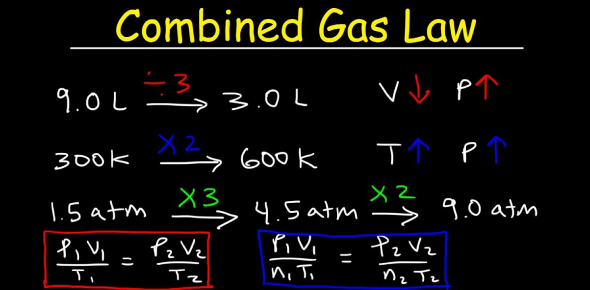# Combined Gas Law Online Quiz

10 Questions | Total Attempts: 2525Settings.

• 1.
If the pressure exerted on a gas is tripled, what will happen to the volume (assuming the temperature and amount of gas remains constant)?
• A.

X2

• B.

X3

• C.

X 1/2

• D.

X 1/3

• 2.
Carbon dioxide occupies a 2.54 L container at STP. What will be the volume when the pressure is 150 KPa and 26 C?
• A.

1.89 L

• B.

42300 L

• C.

0.163 L

• D.

14.1 L

• 3.
In which of the following sets of conditions would a gas most act as a real gas?
• A.

High temperature and low pressure

• B.

High temperature and high pressure

• C.

Low temperature and high pressure

• D.

Low temperature and low pressure

• 4.
Which of the following terms is used to describe the average kinetic energy of the molecules in a sample?
• A.

Manometer

• B.

Temperature

• C.

Pressure

• D.

Volume

• 5.
Which of the following is not a standard pressure measurement?
• A.

101.3 kPa

• B.

1 atm

• C.

760 mm Hg

• D.

273 K

• 6.
The volume of a gas is increased from 150.0 mL to 350.0 mL by heating it. If theoriginal temperature of the gas was 25.0 °C?
• A.

-146 C

• B.

10.7 C

• C.

695 C

• D.

150 C

• 7.
What is the name of the phase change that exists when a solid turns into a liquid?
• A.

Depositon

• B.

Melting

• C.

Sublimation

• D.

Freezing

• 8.
A balloon is inflated to a volume of 130 ml at a pressure of 690 mmHg. If the pressure is increased to 1000 mmHg, what will the new volume be?
• A.

188 ml

• B.

98.8 ml

• C.

89.7 ml

• D.

40300 ml

• 9.
What type of relationship is shown between temperature and pressure?
• A.

Direct

• B.

Indirect (inverse)

• C.

Exponential

• D.

Logarithmic

• 10.
How many mm Hg is equal to 121 kPa?
• A.

1.19

• B.

16.1

• C.

636

• D.

908

Related TopicsBack to top